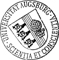## Lower and Upper Bounds for Chord Power Integrals of Ellipsoids

• First we discuss different representations of chord power integrals I_p(K) of any order p >= 0 for convex bodies K (with inner points) in the d-dimensional Euclidean space. Second we derive closed-term expressions of I_p(E(a)) for an ellipsoid E(a) with semi-axes a=(a_1,...,a_d) in terms of the support function of this ellipsoid and prove upper and lower bounds expressed by the volume and the mean breadth of E(a), respectively. A further inequality conjectured for convex body in Davy (1984) is proved for ellipsoids. Some remarks on chord power integrals of superellipsoids and simplices round off the topic. In the Appendix we prove a formula for the (d-1)-volume of (d-1)-ellipsoids arising from the intersection of E(a) with a hyperplane. Further, we derive the exact value of the third-order chord power integral of the Wuerfelecktetraeder correcting a wrong result by Emersleben (1962).

Author: Lothar HeinrichGND urn:nbn:de:bvb:384-opus4-28982 https://opus.bibliothek.uni-augsburg.de/opus4/2898 Preprints des Instituts für Mathematik der Universität Augsburg (2014-07) Preprint English Universität Augsburg 2014/11/19 Poisson cylinder process; integral geometry; convex body; support function; mean breadth; isoperimetric inequality; Carleman's inequality Stochastische Geometrie; Integralgeometrie; Poisson-Prozess; Konvexer Körper; Isoperimetrische Ungleichung Mathematisch-Naturwissenschaftlich-Technische Fakultät Mathematisch-Naturwissenschaftlich-Technische Fakultät / Institut für Mathematik Mathematisch-Naturwissenschaftlich-Technische Fakultät / Institut für Mathematik / Lehrstuhl für Stochastik und ihre Anwendungen 5 Naturwissenschaften und Mathematik / 51 Mathematik / 510 MathematikDeutsches Urheberrecht mit Print on Demand